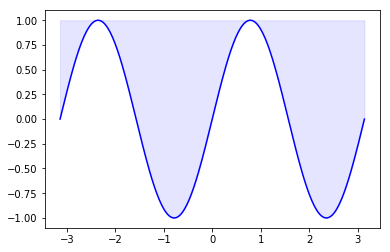## Matplotlib Tutorial

### Matplotlib Object Hierarchy and Important TermsMatplotlib is hierarchically organized. In the previous chapter, we didn't made use of this structure. All we used was a simple plot like plt.plot([2, 5, 9]) which is implicitly building the necessary structures. We didn't have to know about the underlying hierarchical structure. in this chapter of our tutorial we will explain the underlying object hierarchy. We will also demonstrate how to use it to plot graphs and work with the created axes.

A matplotlib object is a tree-like structure of matplotlib objects which build a "hierarchy". The top of the tree-like structure of matplotlib objects is the figure object. A figure can be seen as the container which contains one or more plots. The plots are called axes in matplotlib jargon. Axes is the plural of the word "axis", which gives us a misleading idea. We can think about "axes" as a synonym for the word "plot". The following diagram shows a figure with one axes: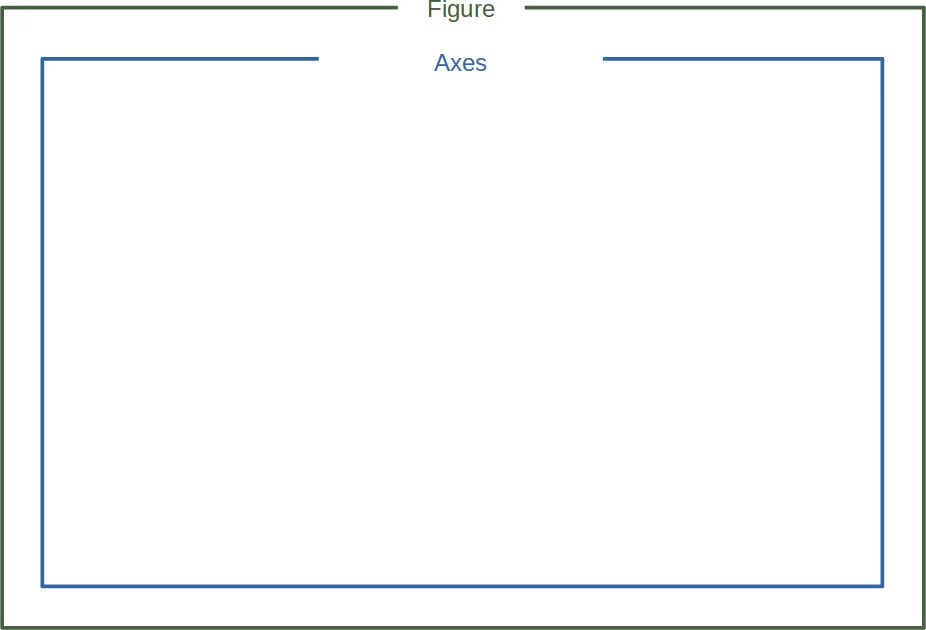As we have already mentioned a figure may also contain more than one axes:The terms axes and figure and in particular the relationship between them can be quite confusing to beginners in Matplotlib. Similarly difficult to access and understand are many of the terms Spine, Tick and Axis. Their function and meaning are easy to crasp, if you see look at them in the following diagram: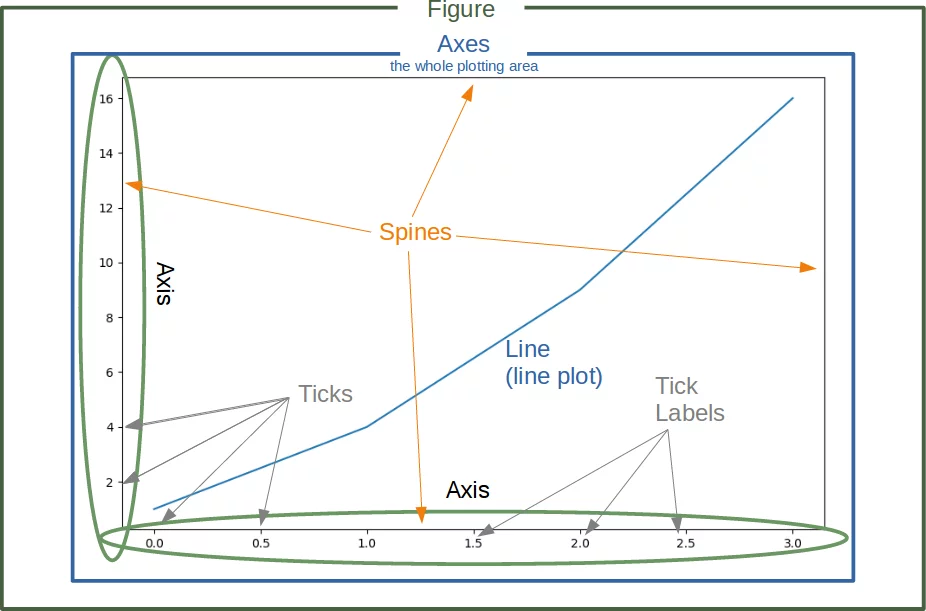### Generate Figure and Axes

import matplotlib.pyplot as plt

fig, ax = plt.subplots()

print(type(fig))
print(type(ax))

<class 'matplotlib.figure.Figure'>
<class 'matplotlib.axes._subplots.AxesSubplot'>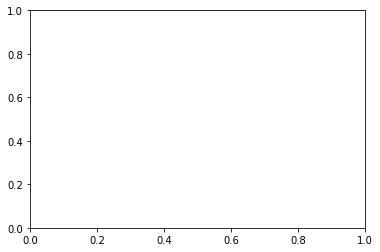The function subplots can be used to create a figure and a set of subplots. In our previous example, we called the function without parameters which creates a figure with a single included axes. we will see later how to create more than one axes with subplots.

We will demonstrate in the following example, how we can go on with these objects to create a plot. We can see that we apply plot directly on the axis object ax. This leaves no possible ambiguity as in the plt.plot approach:

import matplotlib.pyplot as plt

# Data for plotting
X = [2, 4, 6, 8, 10]
Y = [1, 4, 9, 19, 39]

fig, ax = plt.subplots()
ax.plot(X, Y)

Output:
[<matplotlib.lines.Line2D at 0x7f5dbe7b6dd8>]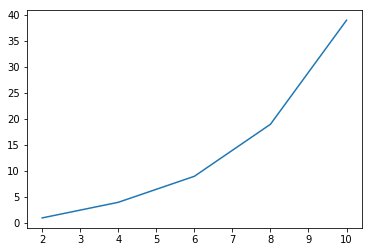### Labels on Axes

We can improve the appearance of our graph by adding labels to the axes. We also want to give our plot a headline or let us call it a title to stay in the terminology of Matplotlib. We can accomplish this by using the set method of the axis object ax:

import matplotlib.pyplot as plt

days = list(range(1,9))
celsius_values = [25.6, 24.1, 26.7, 28.3, 27.5, 30.5, 32.8, 33.1]

fig, ax = plt.subplots()
ax.plot(days, celsius_values)
ax.set(xlabel='Day',
ylabel='Temperature in Celsius',
title='Temperature Graph')

plt.show()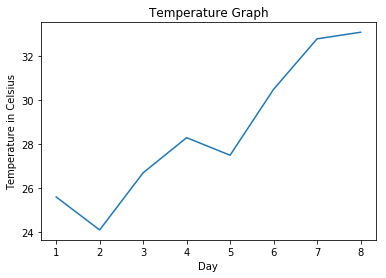### The plot Function

The plot function is needed to plot a figure or better the figures, because there may be more than one. When plot is run in ipython with its pylab mode, it displays all figures and returns to the ipython prompt. When we run it in non-interactive mode, - which is the case, when we run a Python program - it displays all figures and blocks until the figures have been closed.

We can specify an arbitrary number of x, y, fmt groups in a plot function. We will extend our previous temperature example to demonstrate this. We provide two lists with temperature values, one for the minimum and one for the maximum values:

import matplotlib.pyplot as plt

days = list(range(1,9))
celsius_min = [19.6, 24.1, 26.7, 28.3, 27.5, 30.5, 32.8, 33.1]
celsius_max = [24.8, 28.9, 31.3, 33.0, 34.9, 35.6, 38.4, 39.2]

fig, ax = plt.subplots()

ax.set(xlabel='Day',
ylabel='Temperature in Celsius',
title='Temperature Graph')

ax.plot(days, celsius_min,
days, celsius_min, "oy",
days, celsius_max,
days, celsius_max, "or")

plt.show()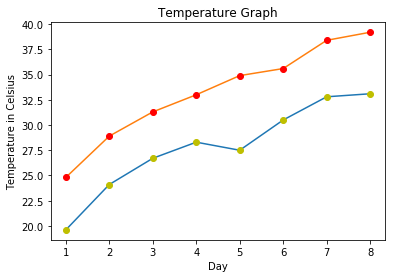We could have used for plot calls in the previous code instead of one, even though this is not very attractive:

import matplotlib.pyplot as plt

days = list(range(1, 9))
celsius_min = [19.6, 24.1, 26.7, 28.3, 27.5, 30.5, 32.8, 33.1]
celsius_max = [24.8, 28.9, 31.3, 33.0, 34.9, 35.6, 38.4, 39.2]

fig, ax = plt.subplots()

ax.set(xlabel='Day',
ylabel='Temperature in Celsius',
title='Temperature Graph')

ax.plot(days, celsius_min)
ax.plot(days, celsius_min, "oy")
ax.plot(days, celsius_max)
ax.plot(days, celsius_max, "or")

plt.show()### Checking and Defining the Range of Axes

We can also check and define the range of the axes with the function axis. If you call it without arguments it returns the current axis limits:

import matplotlib.pyplot as plt

days = list(range(1, 9))
celsius_values = [25.6, 24.1, 26.7, 28.3, 27.5, 30.5, 32.8, 33.1]

fig, ax = plt.subplots()
ax.plot(days, celsius_values)
ax.set(xlabel='Day',
ylabel='Temperature in Celsius',
title='Temperature Graph')

print("The current limits for the axes are:")
print(ax.axis())
print("We set the axes to the following values:")
xmin, xmax, ymin, ymax = 0, 10, 14, 45
print(xmin, xmax, ymin, ymax)
ax.axis([xmin, xmax, ymin, ymax])
plt.show()

The current limits for the axes are:
(0.6499999999999999, 8.35, 23.650000000000002, 33.550000000000004)
We set the axes to the following values:
0 10 14 45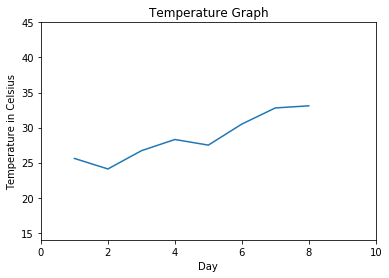### "linspace" to Define X Values

We will use the Numpy function linspace in the following example. linspace can be used to create evenly spaced numbers over a specified interval.

import numpy as np
import matplotlib.pyplot as plt

X = np.linspace(-2 * np.pi, 2 * np.pi, 50, endpoint=True)
F1 = 3 * np.sin(X)
F2 = np.sin(2*X)
F3 = 0.3 * np.sin(X)

fig, ax = plt.subplots()

startx, endx = -2 * np.pi - 0.1, 2*np.pi + 0.1
starty, endy = -3.1, 3.1
ax.axis([startx, endx, starty, endy])

ax.plot(X, F1, X, F2, X, F3)
# discrete points:
ax.plot(X, F1, 'ro', X, F2, 'bx')
plt.show()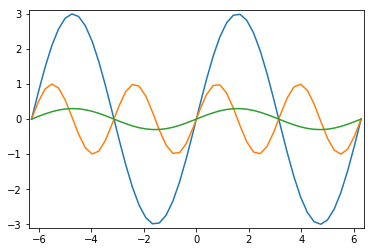### Changing the Line Style

The linestyle of a plot can be influenced with the linestyle or ls parameter of the plot function. It can be set to one of the following values:
'-', '--', '-.', ':', 'None', ' ', ''

We can use linewidth to set the width of a line as the name implies.

import numpy as np
import matplotlib.pyplot as plt

X = np.linspace(0, 2 * np.pi, 50, endpoint=True)
F1 = 3 * np.sin(X)
F2 = np.sin(2*X)
F3 = 0.3 * np.sin(X)
F4 = np.cos(X)

fig, ax = plt.subplots()
ax.plot(X, F1, color="blue", linewidth=2.5, linestyle="-")
ax.plot(X, F2, color="red", linewidth=1.5, linestyle="--")
ax.plot(X, F3, color="green", linewidth=2, linestyle=":")
ax.plot(X, F4, color="grey", linewidth=2, linestyle="-.")
plt.show()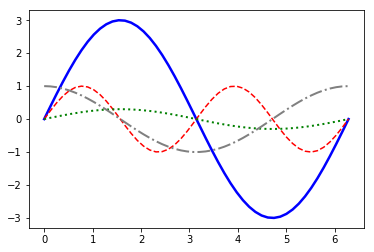### Scatter Plots in Matplotlib

We will learn now how to draw single points in Matplotlib.

import matplotlib.pyplot as plt

fig, ax = plt.subplots()
ax.scatter(3, 7, s=42)

Output:
<matplotlib.collections.PathCollection at 0x7fd1ee805e20>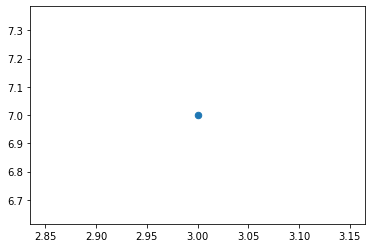import matplotlib.pyplot as plt
import numpy as np

X = np.random.randint(0, 100, (20,))
Y = np.random.randint(0, 100, (20,))
fig, ax = plt.subplots()
ax.scatter(X, Y, s=42)

Output:
<matplotlib.collections.PathCollection at 0x7fd1edfa16d0>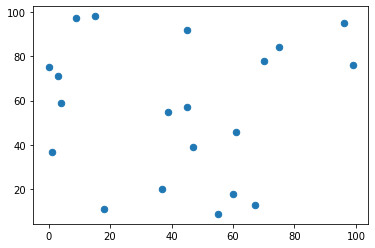## Shading Regions with fill_between()

It is possible to shade or colorize regions between two curves. We are filling the region between the X axis and the graph of sin(2*X) in the following example:

import numpy as np
import matplotlib.pyplot as plt
n = 256
X = np.linspace(-np.pi,np.pi,n,endpoint=True)
Y = np.sin(2*X)

fig, ax = plt.subplots()
ax.plot (X, Y, color='blue', alpha=1.0)
ax.fill_between(X, 0, Y, color='blue', alpha=.2)
plt.show()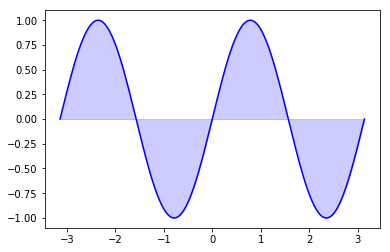The general syntax of fill_between:

fill_between(x, y1, y2=0, where=None, interpolate=False, **kwargs)

The parameters of fill_between:

Parameter Meaning
x An N-length array of the x data
y1 An N-length array (or scalar) of the y data
y2 An N-length array (or scalar) of the y data
where If None, default to fill between everywhere. If not None, it is an N-length numpy boolean array and the fill will only happen over the regions where where==True.
interpolate If True, interpolate between the two lines to find the precise point of intersection. Otherwise, the start and end points of the filled region will only occur on explicit values in the x array.
kwargs Keyword args passed on to the PolyCollection
import numpy as np
import matplotlib.pyplot as plt

n = 256
X = np.linspace(-np.pi,np.pi,n,endpoint=True)
Y = np.sin(2*X)

fig, ax = plt.subplots()

ax.plot (X, Y, color='blue', alpha=1.00)
ax.fill_between(X, Y, 1, color='blue', alpha=.1)
plt.show()